# Solution assignment 10 Optimizing f(x)

### Assignment 10

Find the extreme of the function: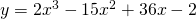without using the sign of the first derivative or the second derivative.

### Solution

In order to find candidates for extremes we have to differentiate the function and make it equal to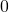: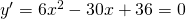thus: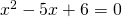This equation has the solutions: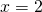or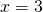A closer look at the cubic equation shows that its graph goes from left under to upper right.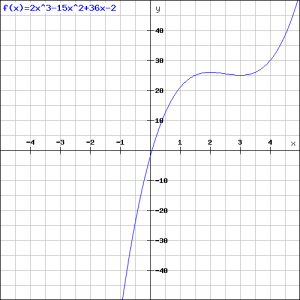For this reason the graph will have a minimum forand a maximum for, see the figure.

0